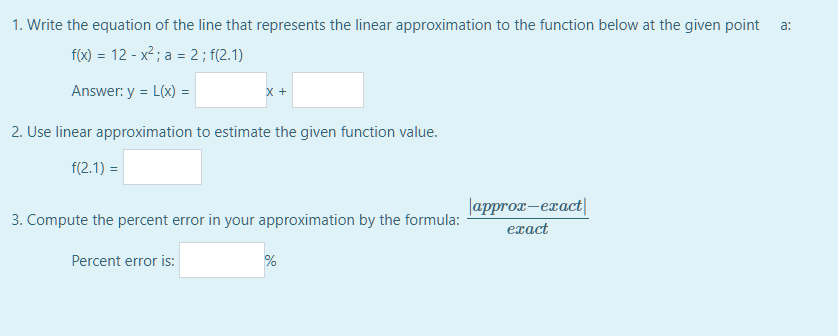what should be put in those blanks?

reopened

 Your name to display (optional): Email me at this address if my answer is selected or commented on: Privacy: Your email address will only be used for sending these notifications. Anti-spam verification: To avoid this verification in future, please log in or register.

(1) f(a)=12-a²; when f(2)=12-4=8. df/dx=-2x=-4 when x=a=2. The point is (2,8) and the gradient at that point is -4, so the linear approximation at (2,8) is L(x)=8-4(x-2)=8-4x+8=16-4x.

Using this approximation we can use it to find f(2.1), which has an actual value of 12-2.1²=7.59.

L(2.1)=16-8.4=7.6.

Error is (7.6-7.59)/7.59=0.01/7.59=0.00132 approx. or 0.13%.

by Top Rated User (1.0m points)
selected by